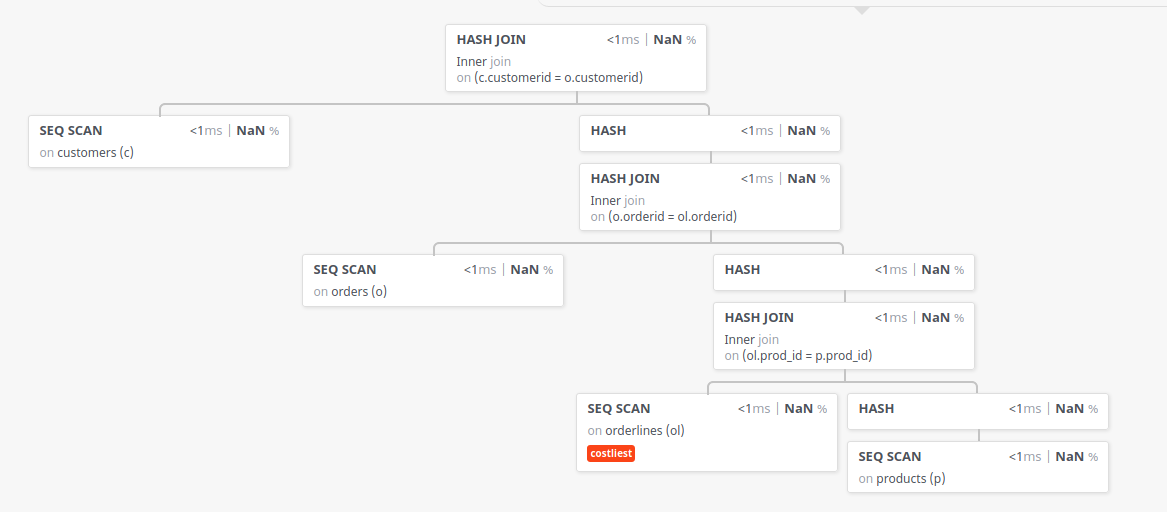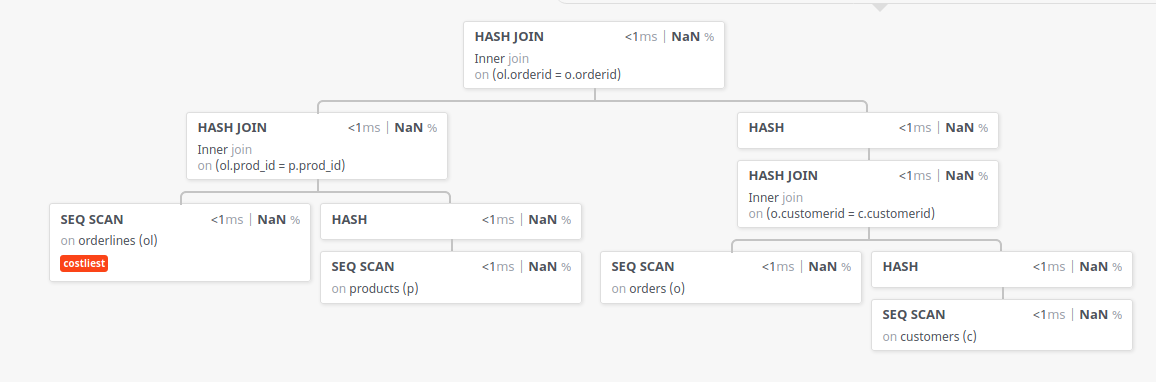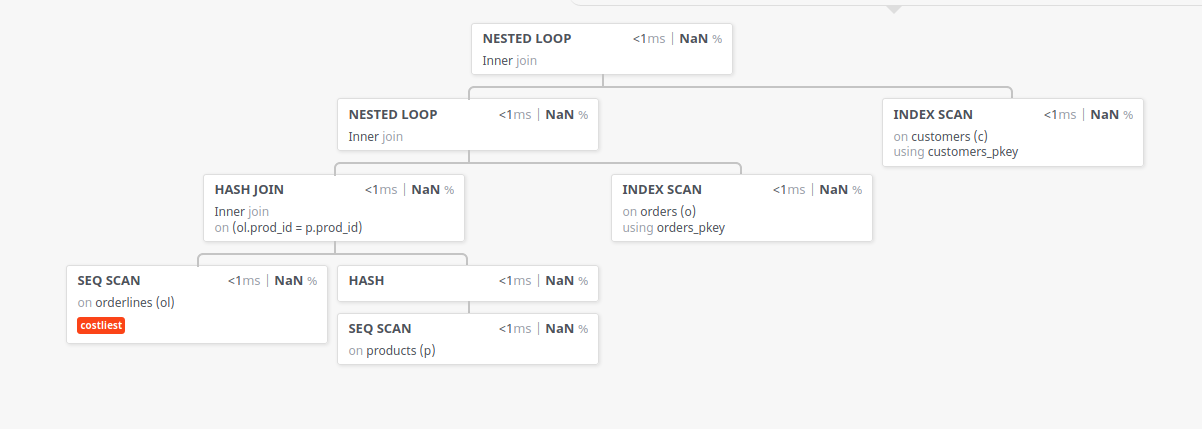## Explain examples

Here are several Dellstore-related queries, with Postgres explain output and also the diagram generated by the site tatiyants.com/pev/#/plans/new. With this information you may be able to figure out how to read the "raw" postgres output.

1. The first query asks for customers who ordered items of cost < 10.0; it comes from exercise 4:

select c.lastname
from customers c join orders o on c.customerid = o.customerid
join orderlines ol on o.orderid = ol.orderid
join products p on ol.prod_id = p.prod_id
where p.price < 10.0;

The diagram generated is as follows (json file here, if you want to try this yourself):What this shows is that the join hierarchy is

customers join (orders join (orderlines join products))

The output of Postgres itself is

QUERY PLAN
--------------------------------------------------------------------------------------------------
Hash Join  (cost=1850.35..2690.87 rows=2752 width=11)
Hash Cond: (c.customerid = o.customerid)
->  Seq Scan on customers c  (cost=0.00..688.00 rows=20000 width=15)
->  Hash  (cost=1815.95..1815.95 rows=2752 width=4)
->  Hash Join  (cost=1508.43..1815.95 rows=2752 width=4)
Hash Cond: (o.orderid = ol.orderid)
->  Seq Scan on orders o  (cost=0.00..220.00 rows=12000 width=8)
->  Hash  (cost=1474.03..1474.03 rows=2752 width=4)
->  Hash Join  (cost=231.70..1474.03 rows=2752 width=4)
Hash Cond: (ol.prod_id = p.prod_id)
->  Seq Scan on orderlines ol  (cost=0.00..988.50 rows=60350 width=8)
->  Hash  (cost=226.00..226.00 rows=456 width=4)
->  Seq Scan on products p  (cost=0.00..226.00 rows=456 width=4)
Filter: (price < 10.0)

This shows the same nesting pattern. There are three Hash Join lines (in bold) generating the same join sequence.

2. The second query is the same as the first, but with a much less selective WHERE clause:

select c.lastname
from customers c join orders o on c.customerid = o.customerid
join orderlines ol on o.orderid = ol.orderid
join products p on ol.prod_id = p.prod_id
where p.price < 16.0;

The diagram output is as follows (json file here)From the diagram we can see a different join pattern: (orderlines join products) join (orders join customers). This structure can also be seen in the textual output of Postgres explain:

QUERY PLAN
------------------------------------------------------------------------------------------
Hash Join  (cost=1740.25..3428.07 rows=19916 width=11)
Hash Cond: (ol.orderid = o.orderid)
->  Hash Join  (cost=267.25..1681.22 rows=19916 width=4)
Hash Cond: (ol.prod_id = p.prod_id)
->  Seq Scan on orderlines ol  (cost=0.00..988.50 rows=60350 width=8)
->  Hash  (cost=226.00..226.00 rows=3300 width=4)
->  Seq Scan on products p  (cost=0.00..226.00 rows=3300 width=4)
Filter: (price < 16.0)
->  Hash  (cost=1323.00..1323.00 rows=12000 width=15)
->  Hash Join  (cost=938.00..1323.00 rows=12000 width=15)
Hash Cond: (o.customerid = c.customerid)
->  Seq Scan on orders o  (cost=0.00..220.00 rows=12000 width=8)
->  Hash  (cost=688.00..688.00 rows=20000 width=15)
->  Seq Scan on customers c  (cost=0.00..688.00 rows=20000 width=15)

The two inner Hash Join lines are not quite lined up, because what Postgres is lining up is the first indented -> Hash Join and the subsequent -> Hash. These identify the respective joins (orderlines join products) and (orders join customers); these two are joined by the topmost join.

This next query comes from Exercise 6:

select c.lastname
from customers c join orders o on c.customerid = o.customerid
join orderlines ol on o.orderid = ol.orderid
join products p on ol.prod_id = p.prod_id
where p.title = 'ACE PIRATES';

The graphical explain output is (json here)The text-based explain output is as follows; the "Nested Loop" means Nested Loop join:

QUERY PLAN
-----------------------------------------------------------------------------------------
Nested Loop  (cost=226.58..1445.79 rows=6 width=11)
->  Nested Loop  (cost=226.30..1442.81 rows=6 width=4)
->  Hash Join  (cost=226.01..1440.88 rows=6 width=4)
Hash Cond: (ol.prod_id = p.prod_id)
->  Seq Scan on orderlines ol  (cost=0.00..988.50 rows=60350 width=8)
->  Hash  (cost=226.00..226.00 rows=1 width=4)
->  Seq Scan on products p  (cost=0.00..226.00 rows=1 width=4)
Filter: ((title)::text = 'ACE PIRATES'::text)
->  Index Scan using orders_pkey on orders o  (cost=0.29..0.31 rows=1 width=8)
Index Cond: (orderid = ol.orderid)
->  Index Scan using customers_pkey on customers c  (cost=0.29..0.49 rows=1 width=15)
Index Cond: (customerid = o.customerid)

Either way, the join order is (((orderlines join products) join orders) join customers)